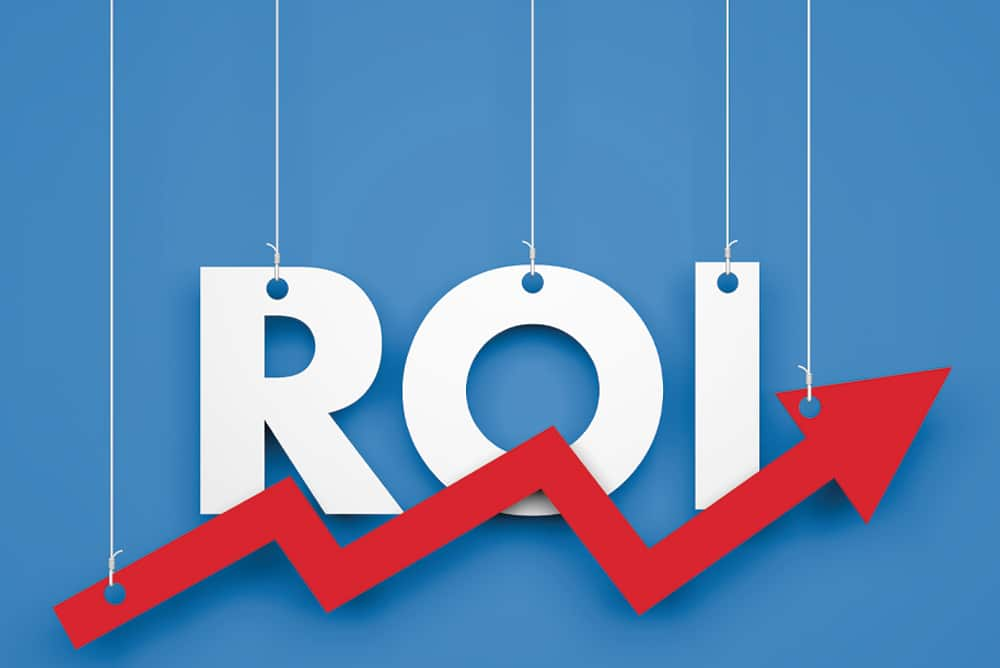Blog Details

# How to Find Your Return on Investment (ROI) in Real EstateYou may have heard of a return on investment (ROI) before, but do you know what it is? The concept of ROI can be very confusing to understand, and there are various ways it can be applied. In this article, we will go over what determines your return on investment, how to calculate your return on investment and some examples of real estate projects with proven high returns.

A lot of people think that real estate is an easy way to make money. But there are a lot of factors involved in making money from real estate, and the return on investment (ROI) is one of them.

The ROI calculation is based on how much you make after expenses and how much income you earn each month from the property. It’s not always easy to calculate your ROI, but if you know how to do it, you can use it to determine whether or not you should buy a property.

You want to find your real estate return on investment (ROI). You want to know whether you are getting a good return on your investment and if you are making money. But how do you calculate ROI?

We calculate ROI by comparing the amount of money we spend on investment with how much it is worth in the future. For example, if we buy a house for \$200,000 and sell it two years later for \$300,000, then our cost of capital is 30% (\$100,000). If we had rented out this same house for \$1,500/month instead of buying it for \$200,000, then our cost of capital would be 25% (\$150/month), which would mean our return on investment would be 125%.

It’s even easier than that! There are several ways to calculate ROI:

Net Present Value (NPV)

An investment's net present value (NPV) is simply the sum of all future cash flows expected to be received by the project, discounted back to today’s value using the appropriate discount rate.

For example, if you are considering purchasing a property for \$100 and expect \$50 in annual rental income over the next five years, your net present value will be \$50 + \$50 = \$100. If you could purchase that property for 0% down and no interest payments during those five years, your return on investment would be 100%.

Cash on Cash Return:

Cash on cash return is the most basic metric to compare different properties, and it’s easy to calculate. The formula is simple: